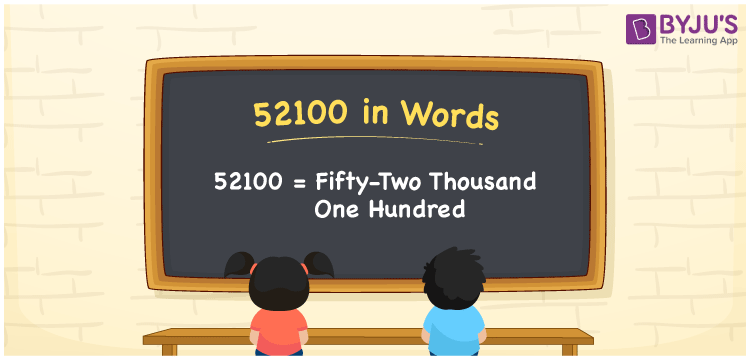52100 in words is written as Fifty Two Thousand One Hundred. In 52100, 5 has a place value of ten thousand, 2 is in the place value of thousand and 1 has the place value of hundred. The article on Place Value gives more information. The number 52100 is used in expressions that relate to money, distance, social media views, and many more. For example, “There were Fifty Two Thousand One Hundred people at a gathering.”

 52100 in words Fifty Two Thousand One Hundred Fifty Two Thousand One Hundred in Numbers 52100

## 52100 in English Words## How to Write 52100 in Words?

We can convert 52100 to words using a place value chart. The number 52100 has 5 digits, so let’s make a chart that shows the place value up to 5 digits.

 Ten thousand Thousands Hundreds Tens Ones 5 2 1 0 0

Thus, we can write the expanded form as:

5 × Ten thousand + 2 × Thousand + 1 × Hundred + 0 × Ten + 0 × One

= 5 × 10000 + 2 × 1000 + 1 × 100 + 0 × 10 + 0 × 1

= 52100.

= Fifty Two Thousand One Hundred.

52100 is the natural number that is succeeded by 52099 and preceded by 52101.

52100 in words – Fifty Two Thousand One Hundred.

Is 52100 an odd number? – No.

Is 52100 an even number? – Yes.

Is 52100 a perfect square number? – No.

Is 52100 a perfect cube number? – No.

Is 52100 a prime number? – No.

Is 52100 a composite number? – Yes.

## Solved Example

1. Write the number 52100 in expanded form

Solution: 5 x 10000 + 2 x 1000 + 1 x 100 + 0 x 10 + 0 x 1

Or Just 5 x 10000 + 2 x 1000 + 1 x 100

We can write 52100 = 50000 + 2000 + 100 + 00 + 0

= 5 x 10000 + 2 x 1000 + 1 x 100 + 0 x 10 + 0 x 1.

## Frequently Asked Questions on 52100 in words

Q1

### How to write the number 52100 in words?

52100 in words is written as Fifty Two Thousand One Hundred.
Q2

### Is 52100 divisible by 3?

No. 52100 is not divisible by 3.
Q3

### Is 52100 divisible by 10?

Yes. 52100 is divisible by 10.